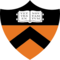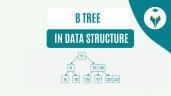Euclidean Algorithm: Method to Find GCD

# Euclidean Algorithm: Method to Find GCD

clickHere
Updated on Aug 31, 2023 10:32 IST

In this article, we will discuss what is Euclidean Algorithm, what is Extended Euclidean Algorithm, and how to use them to find the GCD of two numbers.

Euclidean Algorithm or Euclidean Division Algorithm is a method to find the Greatest Common Divisor (GCD) of two integers. It reduces the problem of finding the GCD of two numbers into smaller and more manageable tasks, and with every iteration, the algorithm brings closer to the solution.

### What is GCD (Greatest Common Divisor)?

The greatest Common Divisor or Highest Common Factor (HCF) of two or more numbers is the greatest common factor that divides each such that the remainder is zero.

Example:

• GCD(10, 7) = 1, where 1 is the largest number that divides both the number.
• GCD (10, 5) = 5, where 5 is the largest number that divides 5 and 10.
• GCD (54, 24) = 6

The factorisation of 54 = 2 x 3 x 3 x 3

The factorisation of 24 = 2 x 2 x 2 x 3

Common Divisor of 54 and 24 is 2 x 3 = 6

Hence, 6 is the GCD of 54 and 24.

#### Methods to find GCD

• Prime Factorization Method
• Long Division Method
• Euclidean Algorithm

In this article, we will explore Euclidean Algorithm Extended Euclidean Algorithm. With the help of examples, we will explain how to use Euclidean Algorithm to find the GCD of two numbers.

But, before starting the article, let’s discuss what this GCD is.

Let’s start the article with the formal definition of Euclidean Algorithm.

## What is Euclidean Algorithm?

Euclidean Algorithm is one of the oldest algorithms that was published around 300 BC which is based on the principle that the GCD of two numbers does not change if the larger number is replaced by its difference with the smaller number, i.e.,

GCD(252, 105) = GCD(252-105, 105) = GCD(147, 105) = 21

### Steps to Find GCD Using Euclidean Algorithm

Let a and b be two numbers such that a > b.

• Divide the larger number a by, the smaller number b
• Replace ‘a’ with ‘b’ and ‘b’ with the remainder from step-1
• Repeat step-1 and step-2 until the remainder is zero.
• Once you get the remainder 0, the divisor will be the GCD of a and b at this stage.

Now, let’s take some examples to understand better how to use the Euclidean Algorithm to find the GCD of two numbers.

### Examples of Euclidean Algorithm

#### Find the GCD of 48 and 18.

48 = 18 * 2 + 12

18 = 12 * 1 + 6

12 = 6 * 2 + 0

Hence, the GCD(48, 18) = 6.

#### Use Euclidean Algorithm to find HCF of 1190 and 1445.

1445 = 1190 * 1 + 255

1190 = 255 * 4 + 170

255  = 170 * 1 + 85

170  = 85 * 2 + 0

Hence, the HCF of 1190 and 1445 is 85.

#### Use Euclidean Algorithm to find HCF of 4052 and 12576.

12576 = 4052 * 3 + 420

4052  = 420 * 9 + 272

420   = 272 * 1 + 148

272   = 148 * 1 + 124

148   = 124 * 1 + 24

124   = 24 * 5 + 4

24    = 4 * 6 + 0

Hence, the HCF of 12576 and 4052 is 4.

## What is an Extended Euclidean Algorithm?

The Extended Euclidean Algorithm is an extension of the Euclidean Algorithm that computes the GCD of two numbers and finds a way to represent the GCD as a combination of two integer coefficients.

i.e. for any integer a and b, there exists x and y such that

GCD(a, b) = a*x + b*y, where

x and y are also an integer, and these coefficients are termed Bezout’s Identity.

Now, let’s take some examples to understand better how to use the Euclidean Algorithm to find the GCD of two numbers.

Find Bezout’s Identities for two numbers, 48 and 18 using Extended Euclidean Algorithm.

Firstly, we will find the GCD using Euclidean Algorithm.

48 = 18 x 2 + 12

18 = 12 x 1 + 6

12 = 6 x 2 + 0

Now, using the back substitution, we will find the coefficients:

6 = 18 – 12 * 1

=> 6 = 18 – (48 – 18*2) * 1 = 18 – 48 + 18*2 = 48*(-1) + 18*(3)

=> 6 = 48*(-1) + 18*(3)

Hence, the Bezouts’ Identities are -1 and 3.

## How to Find the GCD of Two Numbers Using Euclidean Algorithm in Python?

` `
```# define a function to find the GCD using Euclidean Algorithm def gcd(a, b):    # If the second number is 0, the first number is the GCD    if b == 0:        return a    else:        # Recursively call the function with 'b' and the remainder of 'a' divided by 'b'        return gcd(b, a % b)Copy code```

Now, let’s use the same above example to verify the results.

Example-1:

` `
```# Find the GCD of 48 and 18. gcd(48,18)Copy code```

Output

Example-2:

` `
```# Use Euclidean Algorithm to find HCF of 1190 and 1445. gcd (1445, 1190)Copy code```

Output

Exmaple-3:

` `
```# Use Euclidean Algorithm to find HCF of 4052 and 12576. gcd (12576, 4052)Copy code```

Output

## Time and Space Complexity of Euclidean Algorithm

Time Complexity: O(log min(a,b))

Space Complexity: O(1)

Explanation for Time Complexity: At each step, one of the two numbers is reduced to the remainder of the division of the two numbers, which is, at most, half of the smaller number. Therefore the size of numbers decreases exponentially, leading to a logarithmic number of steps. This makes the algorithm extremely efficient, as the number of operations grows slowly with the input size.

Explanation for Space Complexity: The algorithm uses a constant amount of time since it only needs to store a few variables at any given time, i.e., a, b, and the remainder of the division. The space doesn’t increase with the size of the input.

## Conclusion

This article briefly discussed the Euclidean theorem, the Extended Euclidean theorem and how to use them to find the GCD and LCM of two numbers. Hope you will like the article.

Keep Learning!!

Keep Sharing!!

## FAQs

What is Greatest Common Divisior?

The greatest Common Divisor or Highest Common Factor (HCF) of two or more numbers is the greatest common factor that divides each such that the remainder is zero.

What is Euclidean Algorithm?

Euclidean Algorithm is one of the oldest algorithms that was published around 300 BC which is based on the principle that the GCD of two numbers does not change if the larger number is replaced by its difference with the smaller number.

What is Extended Euclidean Algorithm?

The Extended Euclidean Algorithm is an extension of the Euclidean Algorithm that computes the GCD of two numbers and finds a way to represent the GCD as a combination of two integer coefficients.

clickHere

Vikram has a Postgraduate degree in Applied Mathematics, with a keen interest in Data Science and Machine Learning. He has experience of 2+ years in content creation in Mathematics, Statistics, Data Science, and Mac... Read Full Bio

## Trending Technology CoursesData Structures
Coursera4.1Data Structures and Algorithms (III)
Coursera4.0Starts today## Top Picks & New Arrivals